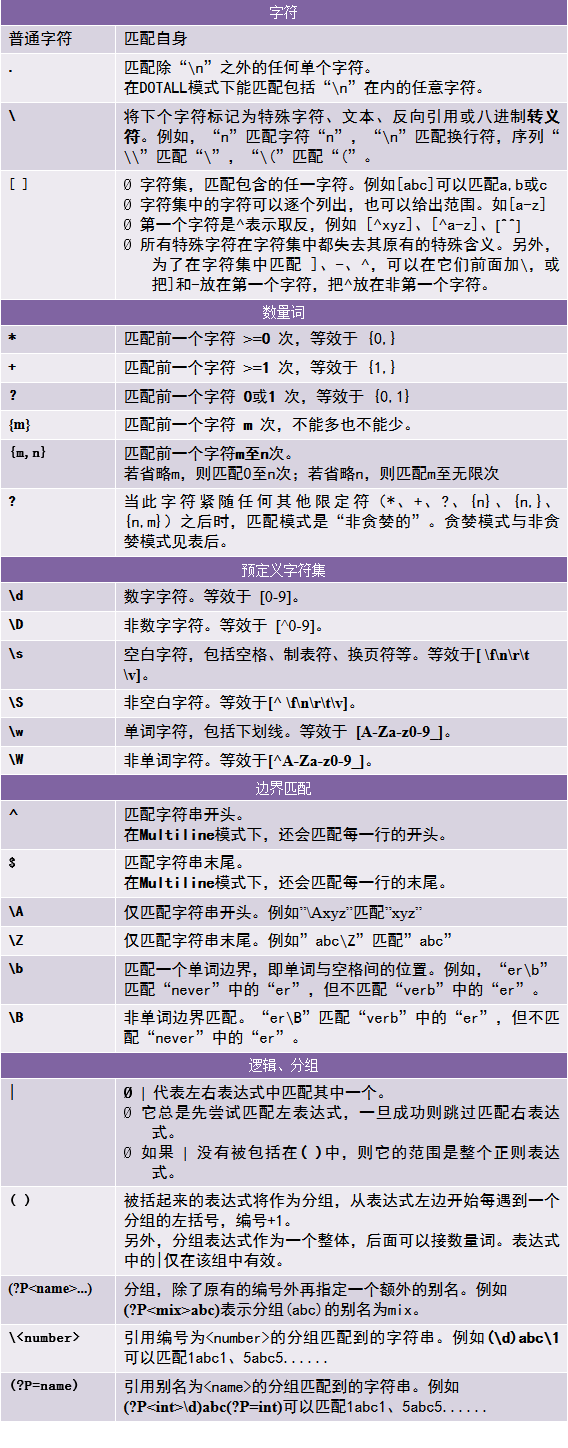一、正则表达式介绍

1）用途

• 测试字符串内的模式。—— 例如，可以测试输入字符串，以查看字符串内是否出现电话号码模式或信用卡号码模式。这称为数据验证。

• 替换文本。—— 可以使用正则表达式来识别文档中的特定文本，完全删除该文本或者用其他文本替换它。

• 基于模式匹配从字符串中提取子字符串。—— 可以查找文档内或输入域内特定的文本。

2）语法3）贪婪模式与非贪婪模式

“贪婪模式”总是尝试匹配尽可能多的字符；“非贪婪模式”则相反，总是匹配尽可能少的字符。例如，用"ab*"如果用于查找"abbbc"，将找到"abbb"。而如果使用非贪婪的数量词"ab*?"，将找到"a"。

二、使用 re 模块

1）编译正则表达式

re 模块提供了 re.compile() 函数将一个字符串编译成 pattern object，用于匹配或搜索。函数原型如下：

re.compile(pattern, flags=0)
re.compile() 还接受一个可选的参数 flag，用于指定正则匹配的模式。关于匹配模式，后面将会讲到。

p = re.compile('ab*', re.IGNORECASE)

3）pattern object 执行匹配

• 匹配从 pos 到 endpos 的字符子串的开头。匹配成功返回一个 match object，不匹配返回 None。
• pos 的默认值是0，endpos 的默认值是 len(string)，所以默认情况下是匹配整个字符串的开头。
import re
pattern = re.compile("ar{1}")
print(pattern.match("army"))     # "ar"在开头，匹配成功
print(pattern.match("mary"))     # "ar"不在开头，匹配失败
print(pattern.match("mary", 1))  # "ar"不在开头，但在子串的开头

# 输出结果：
# <_sre.SRE_Match object; span=(0, 2), match='ar'>
# None
# <_sre.SRE_Match object; span=(1, 3), match='ar'>
• 扫描整个字符串，并返回它找到的第一个匹配（Match object）。
• 和 regex.match() 一样，可以通过 pos 和 endpos 指定范围。
pattern = re.compile("ar{1}")
match = pattern.search("mary")   # search
print(match)

# 输出结果：
# <_sre.SRE_Match object; span=(1, 3), match='ar'>
• 找到所有匹配的子串，并返回一个 list 。
• 可选参数 pos 和 endpos 和上面一样。
pattern = re.compile(r"\d+")
lst = pattern.findall("abc1def2rst3xyz")   # findall
print(lst)

# 输出结果：
# ['1', '2', '3']
• 找到所有匹配的子串，并返回由这些匹配结果（match object）组成的迭代器。
• 可选参数 pos 和 endpos 和上面一样。
pattern = re.compile(r"\d+")
p = pattern.finditer("abc1def2rst3xyz")
for i in p:
print(i)

# 输出结果：
# <_sre.SRE_Match object; span=(3, 4), match='1'>
# <_sre.SRE_Match object; span=(7, 8), match='2'>
# <_sre.SRE_Match object; span=(11, 12), match='3'>

4）match object 获取结果

match object 提供了一些方法，下面列举几个常用的方法，更多请看这里

• 返回 match object 中的字符串。
• 每一个 ( ) 都是一个分组，分组编号从1开始，从左往右，每遇到一个左括号，分组编号+1。
• 组 0 总是存在的，它就是整个表达式 。
• 没有参数时，group1默认为0，这时返回整个匹配到的字符串。
• 指定一个参数（整数）时，返回该分组匹配到的字符串。
• 指定多个参数时，返回由那几个分组匹配到的字符串组成的 tuple。
pattern = re.compile(r"(\w+) (\w+)")
m = pattern.match("Kobe Bryant, Lakers")
print(m)               # <_sre.SRE_Match object; span=(0, 11), match='Kobe Bryant'>
print(m.group())       # Kobe Bryant
print(m.group(1))      # Kobe
print(m.group(2))      # Bryant
print(m.group(1, 2))   # ('Kobe', 'Bryant')
• 返回由所有分组匹配到的字符串组成的 tuple。
>>> m = re.match(r"(\d+)\.(\d+)", "24.1632")
>>> m.groups()
('24', '1632')
• 没有参数时，返回匹配到的字符串的起始位置。
• 指定参数（整数）时，返回该分组匹配到的字符串的起始位置。
pattern = re.compile(r"(\w+) (\w+)")
m = pattern.match("Kobe Bryant, Lakers")
print(m.start())       # 0
print(m.start(2))      # 5
• 没有参数时，返回匹配到的字符串的结束位置。
• 指定参数（整数）时，返回该分组匹配到的字符串的结束位置。
pattern = re.compile(r"(\w+) (\w+)")
m = pattern.match("Kobe Bryant, Lakers")
print(m.end())       # 11
print(m.end(1))      # 4
• 返回一个二元 tuple 表示匹配到的字符串的范围，即 (start, end)。
• 指定参数时，返回该分组匹配到的字符串的 (start, end)。
pattern = re.compile(r"(\w+) (\w+)")
m = pattern.match("Kobe Bryant, Lakers")
print(m.span())     # (0, 11)
print(m.span(2))    # (5, 11)

5）模块级别的函数

pattern = re.compile(r"(\w+) (\w+)")
m = pattern.match("Kobe Bryant, Lakers")
# 相当于
m = re.match(r"(\w+) (\w+)","Kobe Bryant, Lakers")
re.search(pattern, string, flags=0)

pattern = re.compile(r"(\w+) (\w+)")
m = pattern.search("Kobe Bryant, Lakers")
# 相当于
m = re.search(r"(\w+) (\w+)","Kobe Bryant, Lakers")

6）编译标志（匹配模式）

• re.IGNORECASE：忽略大小写，同 re.I

• re.MULTILINE：多行模式，改变^和\$的行为，同 re.M

• re.DOTALL：点任意匹配模式，让'.'可以匹配包括'\n'在内的任意字符，同 re.S

• re.LOCALE：使预定字符类 \w \W \b \B \s \S 取决于当前区域设定， 同 re.L

• re.ASCII：使 \w \W \b \B \s \S 只匹配 ASCII 字符，而不是 Unicode 字符，同 re.A

• re.VERBOSE：详细模式。这个模式下正则表达式可以是多行，忽略空白字符，并可以加入注释。主要是为了让正则表达式更易读，同re.X。例如，以下两个正则表达式是等价的：

a = re.compile(r"""\d +  # the integral part
\.    # the decimal point
\d *  # some fractional digits""", re.X)
b = re.compile(r"\d+\.\d*")

三、修改字符串

1）分割字符串

split()函数在匹配的地方将字符串分割，并返回一个 list。同样的，re 模块提供了两种 split 函数，一个是 pattern object 的方法，一个是模块级的函数。

• maxsplit用于指定最大分割次数，不指定将全部分割。
pattern = re.compile(r"[A-Z]+")
m = pattern.split("abcDefgHijkLmnoPqrs")
print(m)

# 输出结果：
# ['abc', 'efg', 'ijk', 'mno', 'qrs']
• 模块级函数，功能与 regex.split() 相同。
• flags用于指定匹配模式。
m = re.split(r"[A-Z]+","abcDefgHijkLmnoPqrs")
print(m)

# 输出结果：
# ['abc', 'efg', 'ijk', 'mno', 'qrs']

2）搜索与替换

• 使用 repl 替换 string 中每一个匹配的子串，返回替换后的字符串。若找不到匹配，则返回原字符串。
• repl 可以是一个字符串，也可以是一个函数。
• 当repl是一个字符串时，任何在其中的反斜杠都会被处理。
• 当repl是一个函数时，这个函数应当只接受一个参数（Match对象），并返回一个字符串用于替换。
• count 用于指定最多替换次数，不指定时全部替换。
def fun(m):
return m.group().upper()

pattern = re.compile(r"like", re.I)
s1 = pattern.sub(r"love", "I like you, do you like me?")
s2 = pattern.sub(fun, "I like you, do you like me?")
print(s1)
print(s2)

# 输出结果：
# I love you, do you love me?
# I LIKE you, do you LIKE me?
• 模块级函数，与 regex.sub() 函数功能相同。
• flags 用于指定匹配模式。
• 同 sub()，只不过返回值是一个二元 tuple，即(sub函数返回值, 替换次数)
• 模块级函数，功能同 regex.subn()。